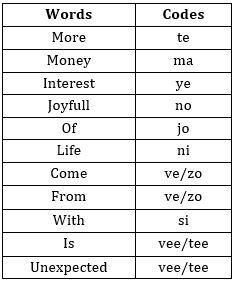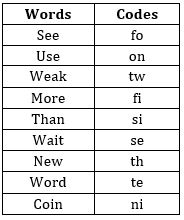Latest Banking jobs   »

# Reasoning Ability Quiz For IBPS RRB PO Clerk Mains 2022- 26th August

Directions (1-5): Study the following information carefully to answer the questions.
In a certain code,
‘Money interest more’ is written as ‘ma te ye’.
‘More of joyfull’ is written as ‘te jo no’.
‘Joyfull come from money’ is written as ‘no ve ma zo’.
‘Life with more of joyfull’ is written as ‘si ni te no jo’.
‘Life is unexpected’ is written as ‘vee ni tee’.

Q1. What is the code for ‘life’?
(a) si
(b) ni
(c) ti
(d) no
(e) Either ‘ni’ or ‘si’

Q2. Which of the following represents ‘life lot of joyfull’?
(a) ma te jo no
(b) si te jo no
(c) te jo no ye
(d) te no jo ke
(e) ni te jo no

Q3. Which of the following may represent ‘life with more of sorrow’?
(a) ni si jo te shi
(b) ni si jo te ve
(c) ni si jo te jo
(d) si ni jo te zo
(e) si ni zo yo ma

Q4. What does ‘zo’ stand for?
(a) Either ‘life’ or ‘with’
(b) Either ‘come’ or ‘from’
(c) Either ‘come’ or ‘with’
(d) Either ‘come’ or ‘life’
(e) None of these

Q5. Which of the following may represent ‘money with more of joyfull’?
(a) si ni te jo ma
(b) te jo ni si ye
(c) si ni te jo no
(d) so si ni jo te
(e) ma si jo no te

Directions (6-10): Answer the questions based on the information given below.
In a certain language,
‘Control beyond world seem’ is coded as ‘R5, H5, H3, R6’
‘During lock period maintain’ is coded as ‘R4, H4, X5, D5’
‘Shall the report execute’ is coded as ‘K3, H5, A4, K5’
‘Without failure done work’ is coded as ‘L5, R3, D4, R4’

Q6. What is the code for ‘JUNIOR’?
(a) N11
(b) R3
(c) Y7
(d) X4
(e) J1

Q7. What is the code for ‘REVIEWING’?
(a) R5
(b) H6
(c) U9
(d) X2
(e) D8

Q8. Which of the following word is coded as ‘X3’?
(a) CUBE
(b) METER
(c) FORD
(d) BRAIN
(e) WASH

Q9. What is the code for ‘COUNT’?
(a) S4
(b) P7
(c) O2
(d) T5
(e) R4

Q10. Which of the following word is coded as ‘H4’?
(a) CUBE
(b) METER
(c) SHALL
(d) STEEL
(e) PAPER

Directions (11-13): Study the following information carefully and answer the questions accordingly.
In an artificial language,
‘Gourmet intrinsic appointed’ is coded as – ‘%L &M &K’
‘Castigate censure blatant’ is coded as – ‘%O %V %Z’
‘Calculate overcome contrive’ is coded as – ‘&E %Z %L’

Q11. What will be the code for ‘Abound announce’?
(a) %V &E
(b) &K %Z
(c) %D &F
(d) &M &Y
(e) None of the above

Q12. Which of the following word will be coded as ‘%Z %L’?
(a) Practical issue
(b) Making formal
(c) Praise problem
(d) Prevent smoke
(e) None of the above

Q13. If the code for ‘Contrive empty’ is ‘%R, ____. Which of the following code will fill the blank space?
(a) %V
(b) %F
(c) &P
(d) &G
(e) None of these

Directions (14-15): Answer the questions based on the information given below.
In a certain code of language,
‘See use weak more’ is coded as ‘tw on fo fi’
‘Wait new than more’ is coded as ‘si fi se th’
‘Use word coin than’ is coded as ‘ni si te on’
‘Weak wait use coin’ is coded as ‘tw on ni se’

Q14. What is the code of ‘coin word’?
(a) on tw
(b) te th
(c) fi si
(d) ni te
(e) None of these

Q15. Which of the following word is coded as ‘tw’?
(a) See
(b) Use
(c) Weak
(d) Than
(e) None of these

SOLUTIONS

Solution (1-5):
Sol.S1. Ans. (b)
S2. Ans. (e)
S3. Ans. (a)
S4. Ans. (b)
S5. Ans. (e)

Solution (6-8):
Sol. The coding language used to code the given words should be decoded in two steps,
Step I: The alphabet is third succeeding alphabet of second alphabet (from left) of the word.
Step II: The number is one plus the number of consonants in the word.
For example: CONTROL is coded as ‘R6’
Alphabet: O + 3 = R
Number: Number of consonants + 1 = 5 + 1 = 6
SO, the code for ‘CUBE’ is X3.
The code for ‘METER’ is ‘H4’
The code for ‘FORD’ is ‘R4’
The code for ‘BRAIN’ is ‘U4’.
The code for ‘WASH’ is ‘D4’ and so on…

S6. Ans. (d)
S7. Ans. (b)
S8. Ans. (a)
S9. Ans. (e)
S10. Ans. (b)

S11. Ans. (d)
Sol. Logic: Symbol: (i) If first letter is consonant then % is used
(ii) If the first letter is vowel, then & is used
Letter: Second letter of the word changed to opposite letter
The code for ‘Abound announce’ – ‘&M &Y’

S12. Ans. (b)
Sol. Logic: Symbol: (i) if first letter is consonant then % is used
(ii) if the first letter is vowel, then & is used
Letter: Second letter of the word changed to opposite letter
Note: Such type of questions is solved with the help of elimination method. We must check all the options one by one.
The code for ‘Making formal’ – %Z %L

S13. Ans. (c)
Sol. Logic: Symbol: (i) If first letter is consonant then % is used
(ii) If the first letter is vowel, then & is used
Letter: Second letter of the word changed to opposite letter
The code for ‘Contrive empty’ is ‘%R, &P’.

Solution (14-15):
Sol.S14. Ans. (d)
S15. Ans. (c)#### Congratulations!Download Hindu Review of October 2021: Free PDF